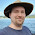## 2012-01-15

Previously observed -- Operations on powers with the same base effectively shift one place down in the order of operations. Examples:
• Exponentiation will multiply powers; e.g., (x^3)^4 = x^12
• Multiplication will add powers; e.g., x^2 * x^3 = x^5
• Addition does a no-op on powers: e.g., 3x^2 + 5x^2 = 8x^2
Note that the same rule generally applies regarding number of places after a decimal point. Examples (working in the other direction):
• Addition does not alter number of places; e.g., 1.2 + 3.4 = 4.6 (1 place)
• Multiplication adds the number of places; e.g., 1.2 * 3.46 = 4.152 (3 places)
• Exponentiation multiplies the number of places; e.g., 1.234^2 = 1.577756 (6 places)
And the reason, of course, is that the decimal places themselves represent exponents to the common base 10. Example:
• 1.2 + 3.4
= (1*10^0 + 2*10^-1) + (3*10^0 + 4*10^-1)
= (1*10^0 + 3*10^0) + (2*10^-1 + 4*10^-1)
= 4*10^0 + 6*10^-1
= 4.6
Since the number of decimal places is dictated by the lowest power, if the addition operation 2*10^-1 + 4*10^-1 doesn't change the power (as above), then neither will it change the number of decimal places. And so on and so forth.

1.1.